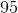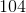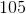# Computer Science : Arrays

## Example Questions

### Example Question #1 : Arrays

int foo[] = {1, 2, 3, 4, 5};

number = 100 + foo;

What is the value of number?

Error.Explanation:

The answer is 105 because arrays are zero indexed. This means that the first position has the subscript 0, the second subscript 1, and so on. 5 is in the fifth space, located at subscript 4. We would access it by saying foo. As another example, if we wanted to access one, we would say foo.

### Example Question #2 : Arrays

Given the following in C++:

int * data = new int;

Pick an expression that is equivalent to : data;

*(data +5);

data + 5

(data+5)*

(data + 5)&

&(data+5)

*(data +5);

Explanation:

Let's look at this line of code:

int * data = new int

An int pointer is created and an array of size 12 is assigned to it.

When data is called, the "data" is dereferenced and the value of the 6th position is returned.

The only one of the choices that does this is

*(data + 5)

In line above, the pointer is incremented ot the 6th position and is then dereferenced to get the value.

If the code was

*data

The first item at the first position will be returned.

*(data+1)

This will return the item and the second position and so on.

# TWO DIMENSIONAL ARRAYS

Given the following initialized array:

int fourth;

int[][] myArray = { {1, 2, 3},

{4, 5, 6},

{7, 8, 9} };

Using myArray, how can I store in variable "fourth", the number 4?

fourth = myArray;

fourth = myArray;

fourth = myArray;

fourth = myArray;

fourth = myArray;

Explanation:

When a two dimensional array is created and initialized, the way to access the items inside the matrix is by calling the array with the row and column (i.e. myArray[ROW][COLUMN]). Keeping in mind that arrays start at 0, the number four would be in row 1, column 0. Therefore to save that number into the variable "fourth" we'll do the following:

fourth = myArray;

*Note: myArray is not the same as myArray.

myArray =4 because it is the item located at row=1 and column = 0.

myArray =12 because it is the item located at row=0 and column = 1.

### Example Question #151 : Computer Science

True or False.

The best data structure to represent a set of keys and values is an array.

False

True

False

Explanation:

Arrays can be two-dimensional. However, when trying to keep track of keys and values it can become complicated when using an array. HashMaps are the best way to represent data containing keys and values.

### Example Question #152 : Computer Science

Define an unwrapped integer array in Swift (iOS).

var arr: [Int] = []

var arr: Int = []

var arr = [Int]

var arr = []

var arr: [Int] = []

Explanation:

In Swift, the variable must be declared first with var then given a name. So now we have var arr then to unwrap, we must add a type var arr: [Int] and then initialize. Therefore, we have var arr: [Int] = []var arr = [] is technically correct, but the prompt asks you to unwrap the variable.

### Example Question #153 : Computer Science

Define an unwrapped string array in Swift (iOS).

var arr = [String]

var arr: String = []

var arr = []

var arr: [String] = []

var arr: [String] = []

Explanation:

In Swift, the variable must be declared first with var then given a name. So now we have var arr then to unwrap, we must add a type var arr: [String] and then initialize. Therefore, we have var arr: [String] = []var arr = [] is technically correct, but the prompt asks you to unwrap the variable.

### Example Question #155 : Computer Science

Which of these instantiate a matrix called matrx with 5 columns and 4 rows that takes in integers?

int [] [] matrx = new int [] ;

int [] [] matrx = new int  ;

int [] [] matrx = new int  ;

int [] [] matrx = new int [] ;

int [] [] matrx = new int  [];